# Electronics and Communication Engineering - Microwave Communication

### Exercise :: Microwave Communication - Section 5

6.

When duct propagation exists

 A. the line of sight and diffraction. Zone concepts are payable B. the line of sight and diffraction zone concepts are not applicable C. line of sight concept is applicable but diffraction zone concept is applicable D. diffraction zone concept is not applicable but line of sight concept is not applicable

Answer: Option B

Explanation:

No answer description available for this question. Let us discuss.

7.

For l << λ, the equivalent circuit of a microwave resistor is

 A.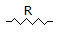B.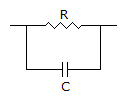C.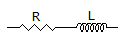D.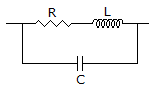Answer: Option B

Explanation:

No answer description available for this question. Let us discuss.

8.

If μr is relative permeability, ∈r is relative permittivity, c is velocity of light fc is cutoff frequency, phase velocity vp (i.e., speed with which a particular phase of the wave travels down a rectangular waveguide at frequency f) is given by

 A.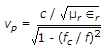B.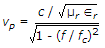C.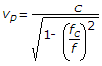D.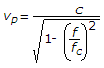Answer: Option A

Explanation:

No answer description available for this question. Let us discuss.

9.

If in line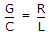the characteristic impedance becomes real.

 A. True B. False

Answer: Option A

Explanation:

No answer description available for this question. Let us discuss.

10.

In a microwave coaxial cable the inner conductor radius is a and inner radius of outside conductor is b. For TE11 mode the cut off wavelength is about

 A. a + b B. p(a + b) C. pa D. pb

Answer: Option B

Explanation:

No answer description available for this question. Let us discuss.

#### Current Affairs 2021

Interview Questions and Answers